# Areas#

This example shows how to create basic geometry using area commands.

```import numpy as np

from ansys.mapdl.core import launch_mapdl

# start MAPDL and enter the pre-processing routine
mapdl = launch_mapdl()
mapdl.clear()
mapdl.prep7()
print(mapdl)
```
```Product:             Ansys Mechanical Enterprise
MAPDL Version:       23.1
ansys.mapdl Version: 0.65.0
```

## APDL Command: A#

Create a simple triangle in the XY plane using three keypoints.

```k0 = mapdl.k("", 0, 0, 0)
k1 = mapdl.k("", 1, 0, 0)
k2 = mapdl.k("", 0, 1, 0)
a0 = mapdl.a(k0, k1, k2)
mapdl.aplot(show_lines=True, line_width=5, show_bounds=True, cpos="xy")
```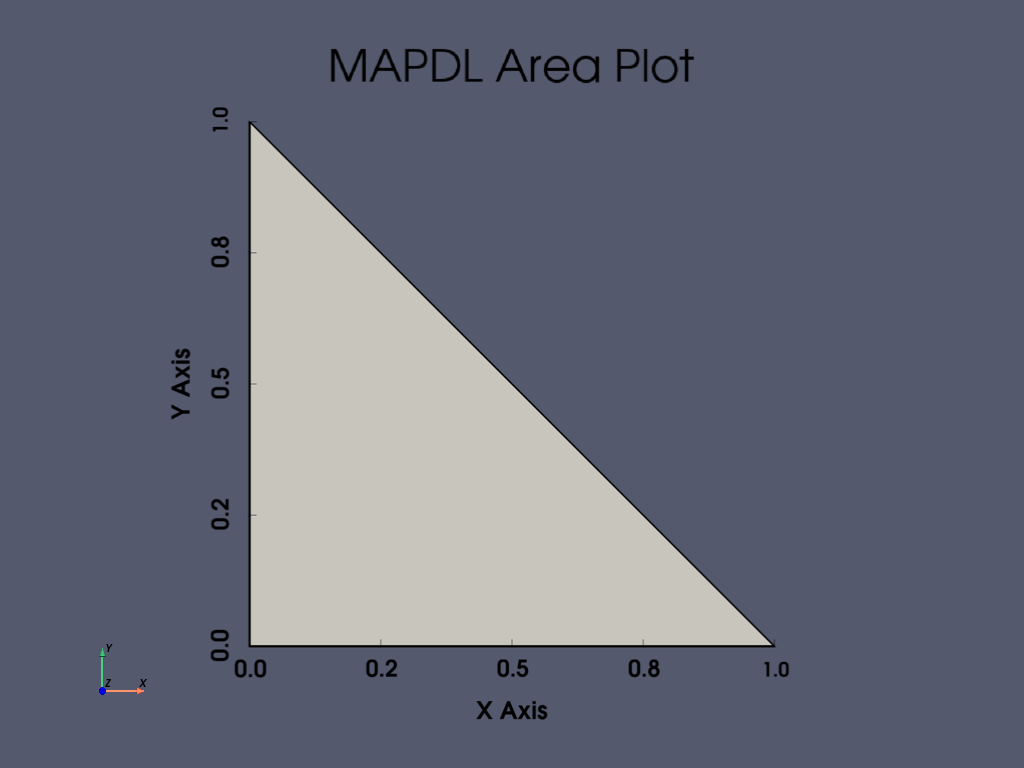## APDL Command: AL#

Create an area from four lines.

```mapdl.clear()
mapdl.prep7()

k0 = mapdl.k("", 0, 0, 0)
k1 = mapdl.k("", 1, 0, 0)
k2 = mapdl.k("", 1, 1, 0)
k3 = mapdl.k("", 0, 1, 0)
l0 = mapdl.l(k0, k1)
l1 = mapdl.l(k1, k2)
l2 = mapdl.l(k2, k3)
l3 = mapdl.l(k3, k0)
anum = mapdl.al(l0, l1, l2, l3)
mapdl.aplot(show_lines=True, line_width=5, show_bounds=True, cpos="xy")
```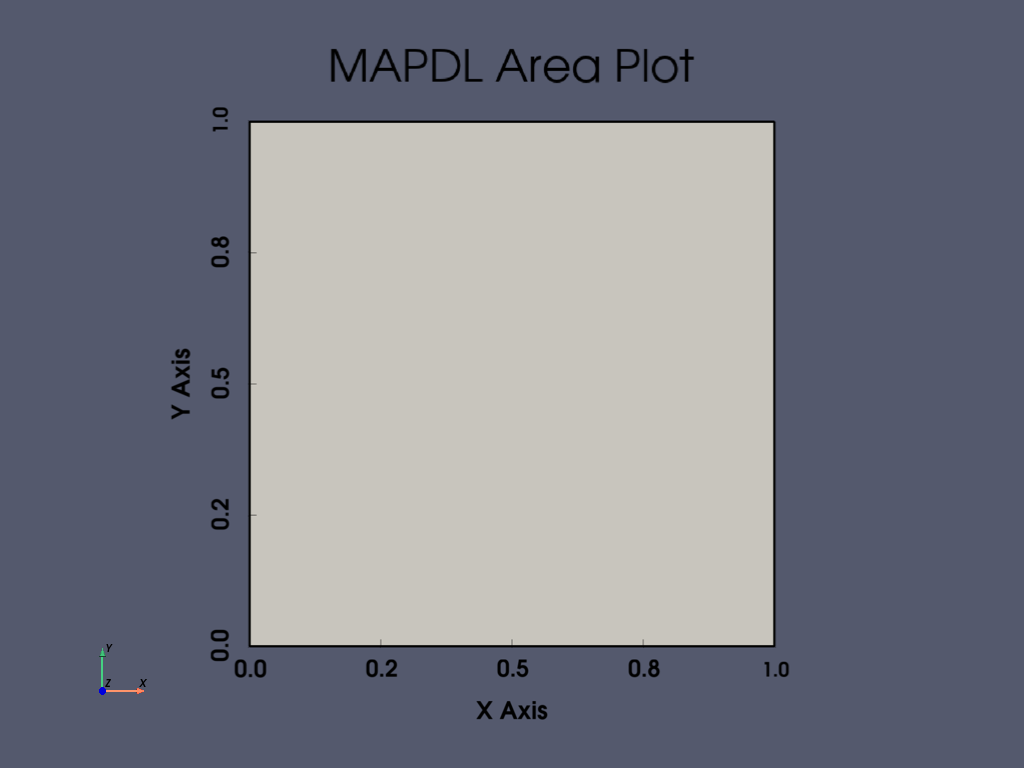Generate areas by dragging a line pattern along a path.

Drag a circle between two keypoints to create an area

```mapdl.clear()
mapdl.prep7()

k0 = mapdl.k("", 0, 0, 0)
k1 = mapdl.k("", 0, 0, 1)
carc = mapdl.circle(k0, 1, k1, arc=90)
l0 = mapdl.l(k0, k1)
```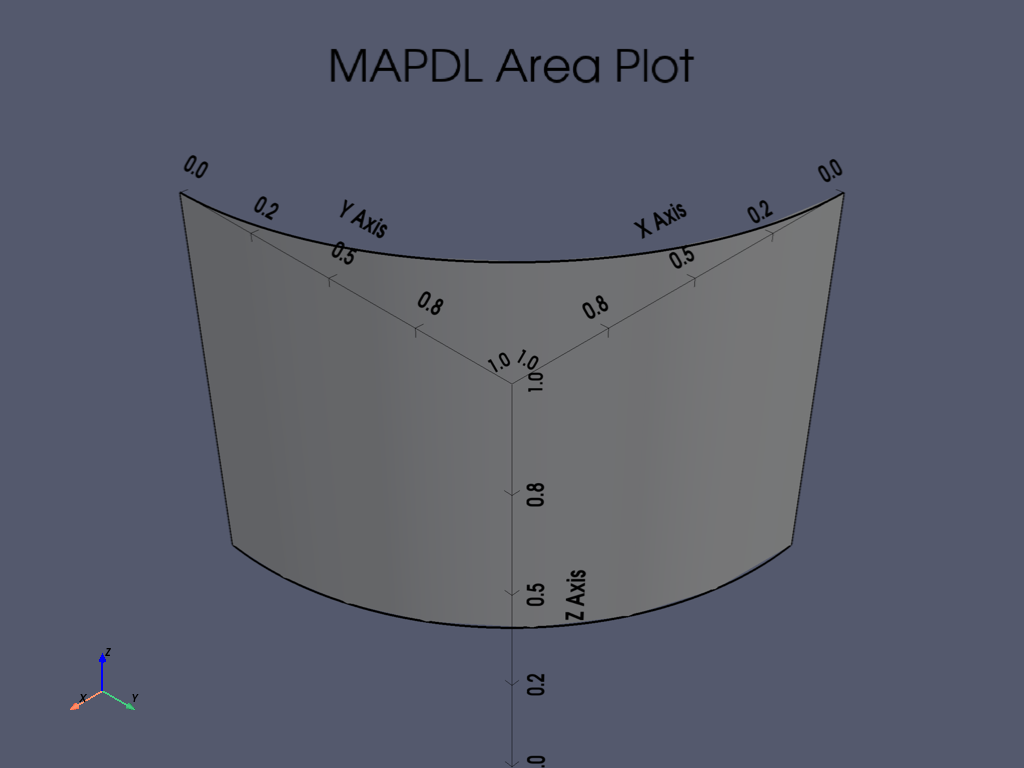## APDL Command: ASBA#

Subtract a `0.5 x 0.5` rectangle from a `1 x 1` rectangle.

```mapdl.clear()
mapdl.prep7()

anum0 = mapdl.blc4(0, 0, 1, 1)
anum1 = mapdl.blc4(0.25, 0.25, 0.5, 0.5)
aout = mapdl.asba(anum0, anum1)
mapdl.aplot(show_lines=True, line_width=5, show_bounds=True, cpos="xy")
```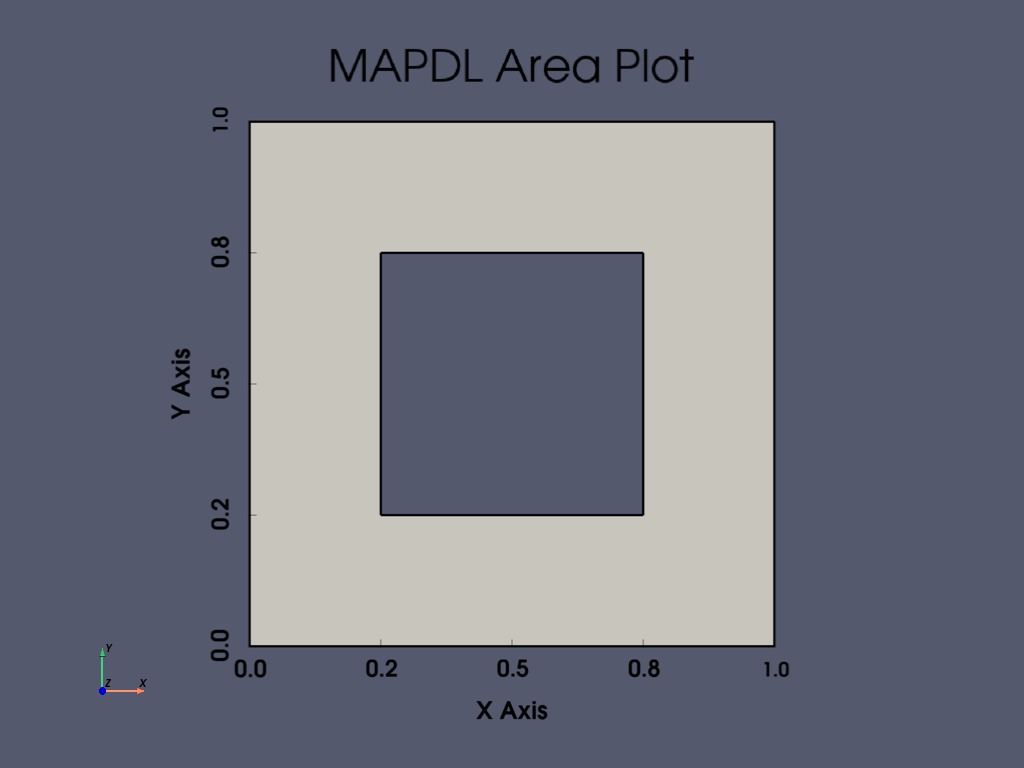## Area IDs#

Return an array of the area IDs

```anum = mapdl.geometry.anum
anum
```
```array(, dtype=int32)
```

## Area Geometry#

Get the VTK `PolyData` containing lines. This VTK mesh can be saved or plotted. For more details, visit Pyvista documentation

Note that this is a method so you can select the quality of the areas (mesh density), and if you would like a merged output or individual meshes.

```areas = mapdl.geometry.areas(quality=3)
areas
```
```[UnstructuredGrid (0x7f38d55ffe80)
N Cells:    12
N Points:   24
X Bounds:   0.000e+00, 1.000e+00
Y Bounds:   0.000e+00, 1.000e+00
Z Bounds:   0.000e+00, 0.000e+00
N Arrays:   3]
```

## Merged Area Geometry#

```area = mapdl.geometry.areas(quality=3, merge=True)
area

# optionally save the area, or plot it
# area.save('mesh.vtk')
# area.plot()
```
PolyDataInformation
N Cells12
N Points24
N Strips0
X Bounds0.000e+00, 1.000e+00
Y Bounds0.000e+00, 1.000e+00
Z Bounds0.000e+00, 0.000e+00
N Arrays1
NameFieldTypeN CompMinMax
entity_numCellsint3213.000e+003.000e+00

## Area Selection#

There are two approaches for selecting areas, the old “legacy” style and the new style. The old style is valuable for those who are comfortable with the existing MAPDL commands, and new style is useful for selecting areas in a pythonic manner.

This example generates a series of random squares and selects them

```mapdl.clear()
mapdl.prep7()

def generate_random_area():
start_x, start_y, height, width = np.random.random(4)
mapdl.blc4(start_x * 10, start_y * 10, height, width)

# create 20 random rectangles
for i in range(20):
generate_random_area()

# Print the area numbers
print(mapdl.geometry.anum)
```
```[ 1  2  3  4  5  6  7  8  9 10 11 12 13 14 15 16 17 18 19 20]
```

Select every other area with the old style command.

```mapdl.asel("S", "AREA", "", 1, 20, 2)
print(mapdl.geometry.anum)
```
```[ 1  3  5  7  9 11 13 15 17 19]
```

Select every other area with the new style command.

Note that the Area IDs are 1 based in MAPDL, while Python ranges are 0 based.

```mapdl.geometry.area_select(range(1, 21, 2))
print(mapdl.geometry.anum)
```
```[ 1  3  5  7  9 11 13 15 17 19]
```

Select areas from a list

Note that you can `return_selected` if you want to see what you have selected. This is helpful when reselecting from existing areas.

```items = mapdl.geometry.area_select([1, 5, 10, 20], return_selected=True)
print(items)
```
```[ 1  5 10 20]
```

## APDL Command: APLOT#

This method uses VTK and pyvista to generate a dynamic 3D plot.

There are a variety of plotting options available for all the common plotting methods. Here, we enable the bounds and show the lines of the plot while increasing the plot quality with the `quality` parameter.

Note that the cpos keyword argument can be used to describe the camera direction from the following:

• `iso` - Isometric view

• `xy` - XY Plane view

• `xz` - XZ Plane view

• `yx` - YX Plane view

• `yz` - YZ Plane view

• `zx` - ZX Plane view

• `zy` - ZY Plane view

```mapdl.aplot(quality=1, show_bounds=True, cpos="iso", show_lines=True)
```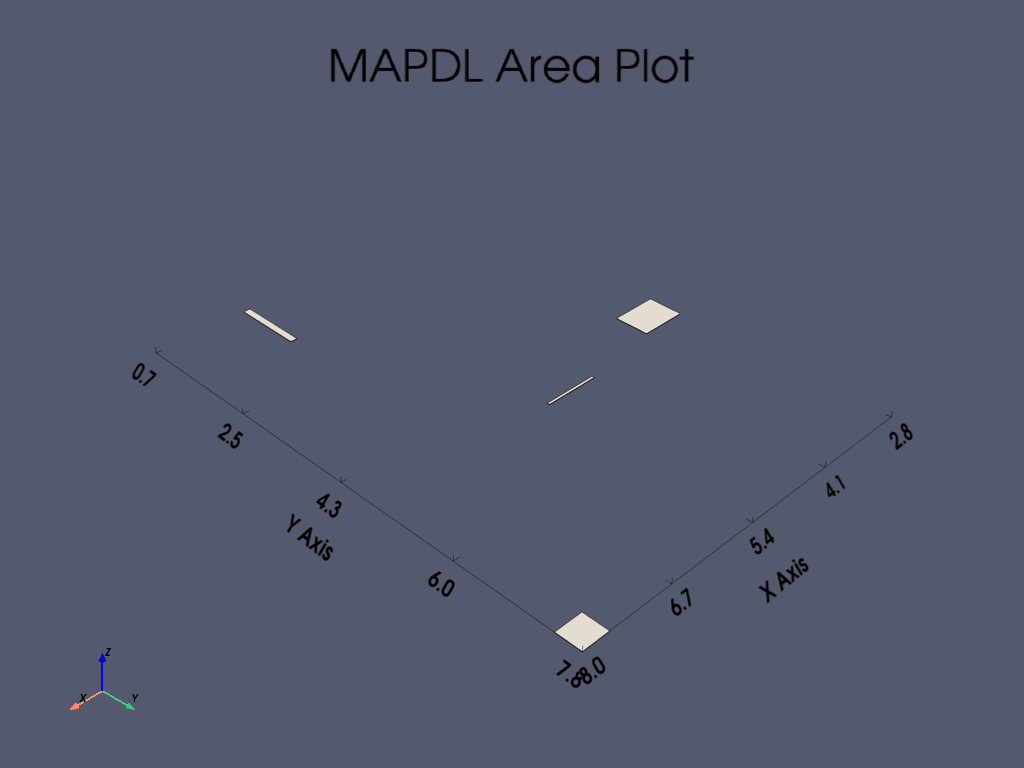## Stop mapdl#

```mapdl.exit()
```

Total running time of the script: ( 0 minutes 4.759 seconds)

Gallery generated by Sphinx-Gallery